Author: Oscar Cronquist Article last updated on May 04, 2022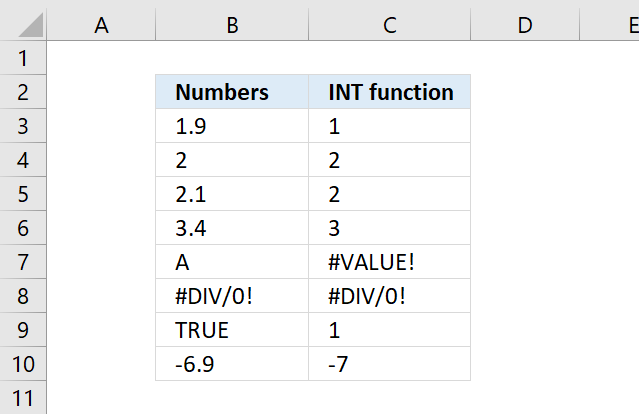The INT function removes the decimal part from positive numbers and returns the whole number (integer) except negative values are rounded down to the nearest integer.

INT(number)

## 2. INT Function Arguments

 number Required. The number you want to convert to an integer.

Boolean values are converted into their equivalent integer. TRUE is 1 and FALSE is 0, see section 5 below for more.

## 3. INT Function ExampleThe image above shows how the INT function handles decimal numbers, cell B3 contains 1.9 and the INT function removes the decimal part. However, this is not the case when a negative number is used. Example, -6.1 is rounded down to -7, see cell C10.

Formula in cell C3:

=INT(B3)

## 4. INT Function alternative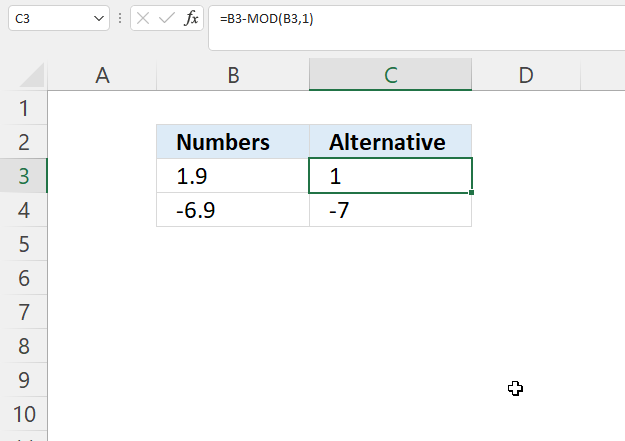Formula in cell C3:

=B3-MOD(B3,1)

### 4.1 Explaining formula

#### Step 1 - Calculate remainder

The MOD function returns the remainder after a number is divided by a divisor.

MOD(numberdivisor)

MOD(B3,1)

becomes

MOD(1.9, 1)

and returns 0.9

#### Step 2 - Subtract the number

The minus sign lets you subtract numbers in an Excel formula.

B3-MOD(B3,1)

becomes

1.9 -0.9 equals 1.

## 5. INT function - convert boolean values to their numerical equivalents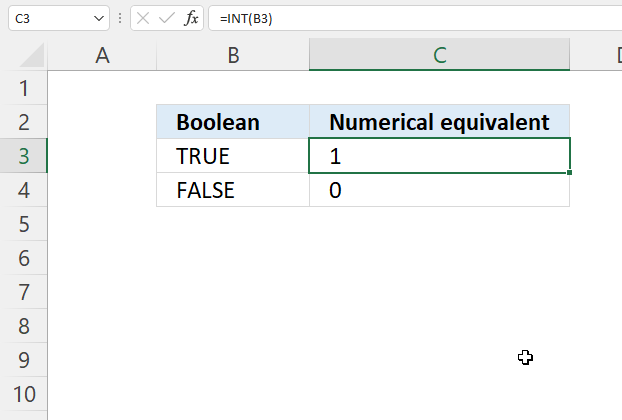The INT function converts a boolean value to its corresponding number.

TRUE - 1
FALSE - 0 (zero)

Formula in cell C3:

=INT(B3)

This can be used in the MMULT function or SUMPRODUCT function to convert boolean values to numerical equivalents. These functions require numerical values to work, there are more ways to convert boolean values:

TRUE + 0 equals 1.
FALSE + 0 equals 0 (zero).

TRUE * 1 equals 1.
FALSE * 1 equals 0 (zero).

## 6. INT function - split string and remove decimals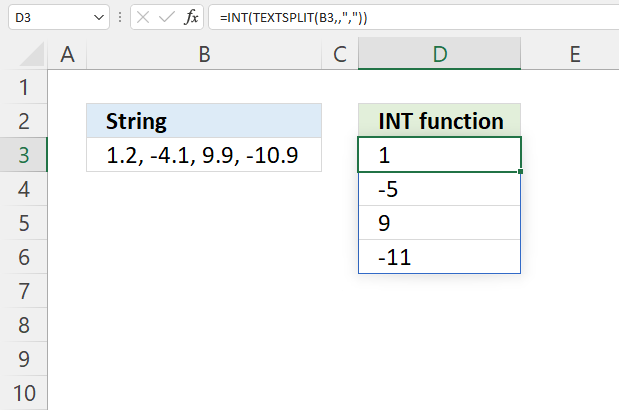Formula in cell D3:

=INT(TEXTSPLIT(B3,,","))

### Explaining formula

#### Step 1 - Split values into an array

The TEXTSPLIT function lets you split a string into an array across columns and rows based on delimiting characters.

TEXTSPLIT(Input_Textcol_delimiter, [row_delimiter], [Ignore_Empty])

TEXTSPLIT(B3,,",")

becomes

TEXTSPLIT("1.2, -4.1, 9.9, -10.9",,",")

and returns

{"1.2";" -4.1";" 9.9";" -10.9"}.

#### Step 2 - Remove decimals

Note that the INT function also converts the text values to numbers automatically.

INT(TEXTSPLIT(B3,,","))

becomes

INT({"1.2"; " -4.1"; " 9.9"; " -10.9"})

and returns {1; -5; 9; -11}. The double quotes are gone indicating they are now numbers and not text.

## 7. INT function and IF function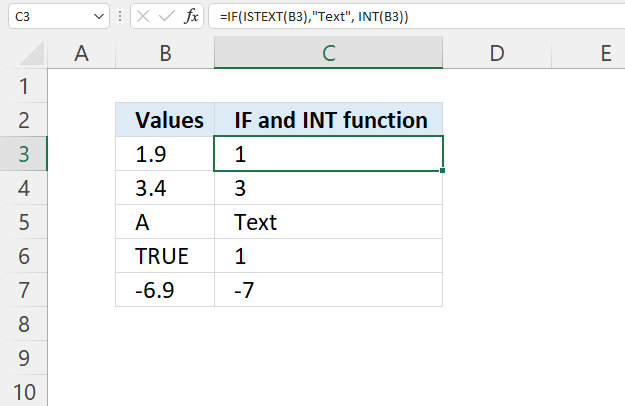This formula checks if the value on the same row in column B is a text value and returns "Text" if so.

Formula in cell C3:

=IF(ISTEXT(B3),"Text", INT(B3))

### 7.1 Explaining formula

#### Step 1 - Check if the value is a text value

The ISTEXT function returns TRUE if the value is a text value.

ISTEXT(value)

ISTEXT(B3)

becomes

ISTEXT(1.9)

and returns FALSE. 1.9 is not a text value.

#### Step 2 - Evaluate IF function

The IF function returns one value if the logical test is TRUE and another value if the logical test is FALSE.

IF(logical_test, [value_if_true], [value_if_false])

IF(ISTEXT(B3),"Text", INT(B3))

becomes

IF(FALSE,"Text", INT(B3))

INT(B3)

becomes

INT(1.9)

and returns 1.

## 8. Split date from a date and time value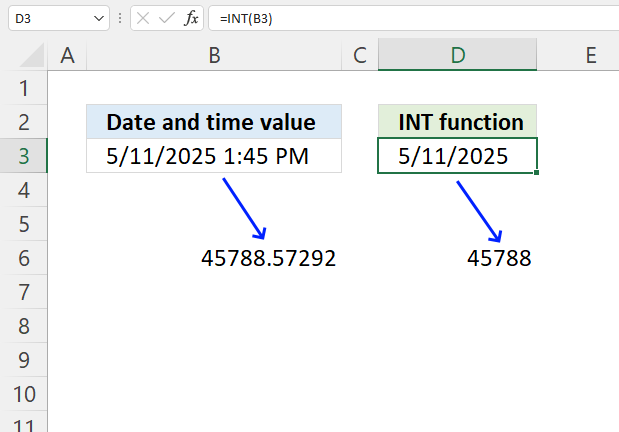The INT function removes the decimals from a number, an Excel date and time value has two parts. The integer is the date and the decimal is the time.

Remove the decimal and the date is left, remove the integer and the time is left.

Formula in cell D3:

=INT(B3)

INT(45788.57292)

returns 45788.

## 9. Split time from a date and time value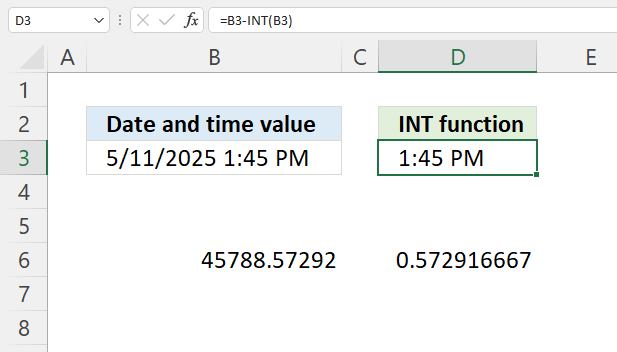This example shows how to extract time from an Excel date and time value.

Formula in cell D3:

=B3-INT(B3)

### Explaining formula

#### Step 1 - Remove decimals

INT(45788.572916667)

returns 45788.

#### Step 2 - Calculate decimals

B3-INT(B3)

becomes

45788.572916667 - 45788

and returns 0.572916667

## 10. Extract the hour from a time value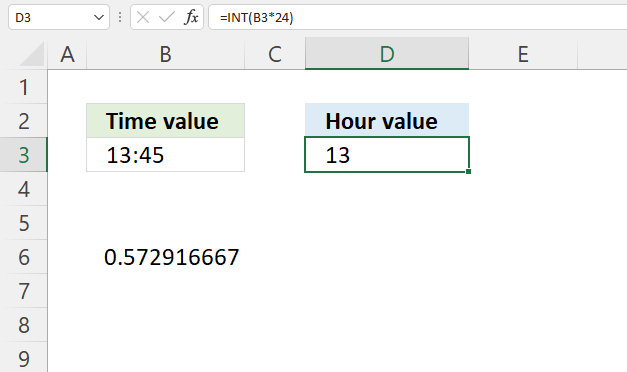The HOUR function lets you calculate the hour value based on an Excel date (and time value), however, you can perform the same calculation using the INT function.

Formula in cell D3:

=INT(B3*24)

### Explaining formula

#### Step 1 - Calculate hours

B3*24

becomes

0.572916666664241*24

and returns

13.7499999999418

#### Step 2 - Remove decimals

INT(B3*24)

becomes

INT(13.7499999999418)

and returns 13.

## 11. Extract the minute from a time value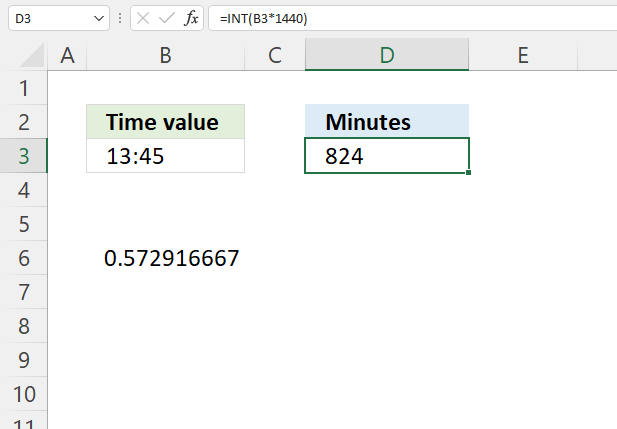Formula in cell D3:

=INT(B3*1440)

### Explaining formula

24 hours contain 1440 minutes. 24*60 = 1440.

B3*1440

INT(B3*1440)